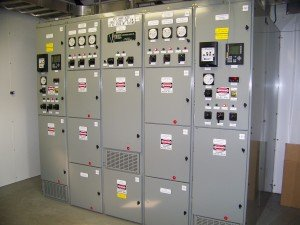## Per Unit and Symmetrical Components – Electrical Power Calculations

Course No.: PSA101
CEUs 1.6

### Overview – Electrical Power Calculations ClassThis course by Jim Phillips, P.E. has become the industry standard that defines the “Crash Course” in electrical power system analysis. Very few universities teach Per Unit and Symmetrical Components calculations anymore but it is still a fundamental concept for electric power system analysis. Jim Phillips teaches you the basics of the per unit system and electric power system analysis during the first day of the two day class.

On the second day, Jim builds upon the per unit system and takes you to the next level with symmetrical components and unbalanced power system calculations. Learn how to more easily use positive, negative and zero sequence components and draw zero sequence diagrams.This class is loaded with many in class examples and problems for a hands on learning experience.

Jim has developed this course based on over 30 years of extensive experience with industrial, commercial and utility power systems.

Training manual and course material
Jim’s calculation worksheets
Many calculation examples and problems
16 hours of Continuing Education Credit

### Have This Class On-Site at Your Location

You can also have this class conducted on-site at your location.  Contact our Program Director at 800.874.8883 to see about having Jim teach this class to your staff at your company’s facilities.  Contact us for your custom on-site training proposal.

### Day One Agenda – Electrical Power Calculations

POWER SYSTEM ANALYSIS CONCEPTS
Introduction to Power System Analysis, Basic Fundamentals, Resistance, Inductance and Capacitance, RLC Circuits, Series and Parallel Circuits, Delta/Wye Conversions, Complex Impedances, Per Phase Analysis, Thevenin Equivalent Impedance

PER UNIT ANALYSIS
Understanding the Per Unit System and Its Uses.  Defining Base Quantities, Per Unit Voltage Per Unite Current and Per Unit Impedance, Selecting the Appropriate MVA and kV Base, Calculating the Base Current and Impedance, Changing Bases, Per Unit Problem Solving Worksheets, Per Unit Calculation Examples and Problems

PER UNIT IMPEDANCE DIAGRAMS
Developing the Per Unit Impedance Diagram, Converting circuit impedances from ohms to per unit values,  Labeling the Diagram, Load Calculations using the Per Unit Diagram Impedance Diagram, Per Unit Short Circuit Calculations using the Impedance Diagram

METERING
Ammeter, Voltmeter, Wattmeter, Varmeter, Current Transformers and Potential Transformers

TRANSFORMERS
Transformer Turns Ratio, Transformer Analysis, Efficiency, Transformer Impedance, Transformer Circuit Model, Modeling Core and Winding Losses, Calculating Voltage Regulation

ROTATING MACHINES
Induction Machines Modeling, Induction Machine Circuit Analysis, Stator and Rotor Impedances, Motor Slip, Poles and Number of Windings, Motor Speed, Calculating Full Load Amps, Locked Rotor Amps and Power Factor. Conversion from Electrical Power to Mechanical Power, Motor Efficiency, Solving induction machine problems

### Day Two Agenda – Electrical Power Calculations

CONDUCTOR MODELING
Impedance Data, Geometric Mean Radius, Geometric Mean Distance, Skin Effect, ACSR and other types of Conductors

TRANSMISSION LINES
Short, Medium and Long Transmission Line Models, Pi and T models.  Effect of Capacitive Line Charging on Longer Lines, Voltage Drop, Sending End and Receiving End Voltage Calculations, Conductor Data a.k.a. “The Birds” Wax Wing, Blue Jay, Partridge etc.

MOTOR STARTING
Using the Per Unit System to Solve Motor Starting Problems, Effect of Source Strength and Motor Starting Current on Voltage Flicker, Electric Utility Concerns, Voltage Flicker Curve, Voltage Flicker Analysis

INTRODUCTION TO SYMMETRICAL COMPONENTS
Introduction to Symmetrical Components, Using Sequence Networks, Positive, Negative and Zero Sequence, Solving Unbalanced Problems Using Three Balanced Set of Vectors, Creating Zero Sequence Diagrams, Effect of Transformer and System Grounding on Zero Sequence Impedance

SYMMETRICAL COMPONENTS CALCULATIONS
Solving Symmetrical Component Problems Painlessly! Transmission Line Calculation Problems and Examples, Solving for Three Phase and Line-to-Ground Short Circuit Current Using Positive, Negative and Zero Sequence Impedances, Example Problem of Ungrounded Transformer’s Effect on Z0

SHORT CIRCUIT CALCULATIONS
Ohmic and Per Unit Calculations, Symmetrical Component Calculations for Balanced and Unbalanced Calculations Using Z1, Z2 and Z0

POWER FACTOR AND HARMONICS
Vars, Watts, VA and Power Factor, Reactive Power and Sizing the Power Factor Correction Capacitor, Harmonic Concerns

Attend This Class to See How To:Perform Calculations with the Per Unit System
Draw Impedance Diagrams
Understand the Selection of Base Values
Properly Use Positive, Negative and Zero Sequence Impedances
Use Jim’s Short Cut Method to Develop Sequence Diagrams
Perform Calculations with Symmetrical Components
Perform Other Types of Calculations such as Power Factor

### Questions?For questions, registration information or to discuss holding this class at your location as an on-site training program, contact our Program Director at 800.874.8883

Brainfiller, Inc. | P.O. Box 12024 | Scottsdale, AZ 85267